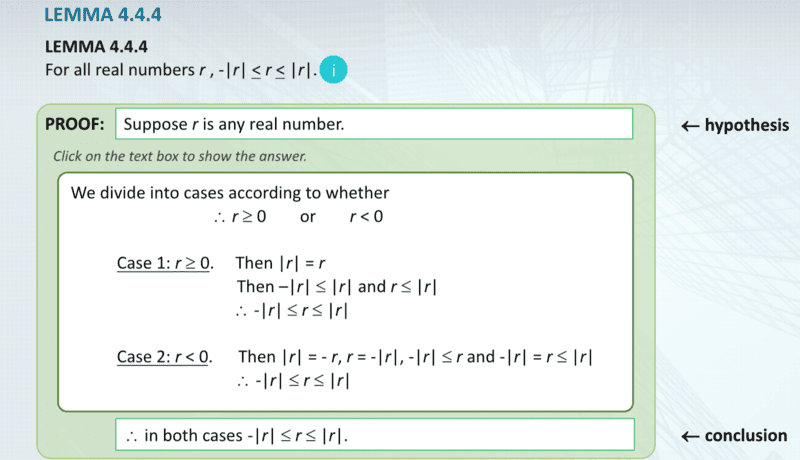# Proof of a Lemma regarding absolute values

• I
• WWCY

#### WWCY

Hi all,

There's this proof that I've been trying to wrap my head around but it just doesn't seem to sink in. I've attached a screenshot below. Many thanks in advance!Consider Case 1. There is a step that goes
$$\text{Then} \ |r| = r$$
$$Then -|r| \leq |r| \ \text{and} \ r \leq |r|$$
Why is this the case? This seems to imply that because ##|r|=r##, then ##r \leq |r|##. Is this because of the "generalisation" rule of inference that goes
$$p$$
$$\text{Therefore} \ p \vee q$$
Where ##p = |r| = r## and ##q = |r| > r##? If so, why not write ##q = |r| < r## and get a completely different result altogether?

#### Attachments

The lemma says ##|r|\leq |r| ## which should be trivial.

For case 1 they already establish ##r=|r| ## so ##r\leq |r|## holds trivially. Yes, ##p\rightarrow p\lor q ## does the trick here.

You may take ##q=|r| ## and then write ##r=|r| \rightarrow r\leq |r| \lor |r|<r ##, but ##q: |r|<r## is simply not true.